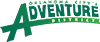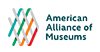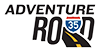• It's Thursday 6:35 PMSorry, we're closed

# Museum Blog

Categorized In:

Categorized In:

Categorized In:

Categorized In:

Categorized In:

Categorized In:

Categorized In:

Categorized In:

Categorized In: ,

Categorized In:

Categorized In:

Categorized In:

Categorized In:

Categorized In:

Categorized In:

Categorized In:

Categorized In:

Categorized In:

Categorized In:

Categorized In:

Categorized In:

Categorized In:

Categorized In:

Categorized In:

Categorized In:

Categorized In:

Categorized In:

Categorized In: ,

Categorized In:

Categorized In:

Categorized In:

Categorized In:

Categorized In:

Categorized In:

Categorized In:

Categorized In:

Categorized In:

Categorized In:

Categorized In:

Categorized In:

Categorized In:

Categorized In:

Categorized In:

Categorized In:

Categorized In:

Categorized In: ,

Categorized In:

Categorized In:

Categorized In: , ,

Categorized In:

Categorized In:

Categorized In:

Categorized In:

Categorized In:

Categorized In: ,

Categorized In:

Categorized In: ,

Categorized In:

Categorized In:

Categorized In:

Categorized In:

Categorized In:

Categorized In: ,

Categorized In:

Categorized In:

Categorized In:

Categorized In: ,

Categorized In:

Categorized In:

Categorized In:

Categorized In:

Categorized In:

Categorized In:

Categorized In:

Categorized In: ,

Categorized In: ,

Categorized In:

Categorized In:

Categorized In:

Categorized In:

Categorized In:

Categorized In:

Categorized In: ,

Categorized In:

Categorized In:

Categorized In: ,

Categorized In:

Categorized In: ,

Categorized In:

Categorized In:

Categorized In: ,

Categorized In: ,

Categorized In:

Categorized In:

Categorized In:

Categorized In:

Categorized In:

Categorized In:

Categorized In:

Categorized In:

Categorized In:

Categorized In:

Categorized In:

Categorized In:

Categorized In:

Categorized In:

Categorized In:

Categorized In:

Categorized In:

Categorized In:

Categorized In:

Categorized In:

Categorized In:

Categorized In:

Categorized In:

Categorized In:

Categorized In:

Categorized In:

Categorized In:

Categorized In:

Categorized In:

Categorized In:

Categorized In:

Categorized In:

Categorized In:

Categorized In:

Categorized In:

Categorized In:

Categorized In:

Categorized In: ,

Categorized In:

Categorized In:

Categorized In: ,

Categorized In:

Categorized In:

Categorized In:

Categorized In: ,

Categorized In:

Categorized In:

Categorized In: ,

Categorized In:

Categorized In:

Categorized In:

Categorized In:

Categorized In:

Categorized In:

Categorized In: ,

Categorized In:

Categorized In: ,

Categorized In: ,

Categorized In:

Categorized In:

Categorized In:

Categorized In:

Categorized In:

Categorized In:WordPress Image Lightbox